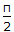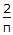# Chemical Engineering - Heat Transfer

### Exercise :: Heat Transfer - Section 11

11.

In a heat exchanger with steam outside the tubes, a liquid gets heated to 45°C, when its flow velocity in the tubes is 2 m/s. If the flow velocity is reduced to 1 m/s, other things remaining the same, the temperature of the exit liquid will be

 A. less than 45°C B. more than 45°C C. equal to 45°C D. initially decreases and remains constant thereafter.

Explanation:

No answer description available for this question. Let us discuss.

12.

For a liquid in laminar flow through a very long tube, when the exit fluid temperature approaches the wall temperature, the equation to be used is

 A. Nu = 0.023 Re0.8 . Pr0.4 B. Nu =Gz C. Nu =Gz D. Nu = 2Gz0.5

Explanation:

No answer description available for this question. Let us discuss.

13.

Multiple effect evaporation accounts for

 A. steam economy B. lower operating costs C. investment economy D. none of these

Explanation:

No answer description available for this question. Let us discuss.

14.

In case of a supercooled solution, which is on the verge of crystallisation, the free energy of the solution as compared to that of the solid is

 A. more B. less C. same D. more or less; depends on the nature of solution

Explanation:

No answer description available for this question. Let us discuss.

15.

Maximum water velocity in tubes of a 1-2 shell and tube heat exchanger may be around __________ metres/second.

 A. 1 B. 10 C. 20 D. 30